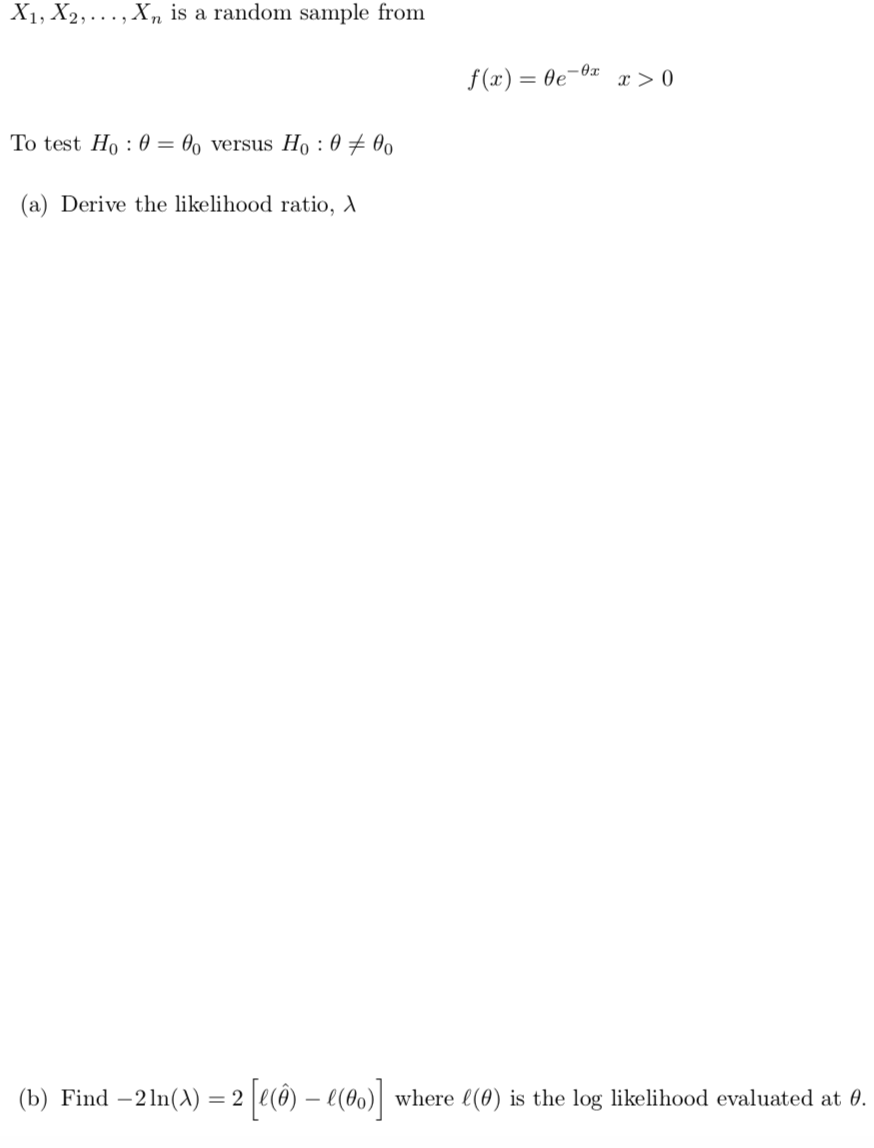# X1, X2, ..., X, is a random sample fromf (x) = 0e-0xx > 0To test Ho : 0 =Oo versus H, : 0 ± 00(a) Derive the likelihood ratio, A(b) Find –2 In(A) = 2 e(ô) – e(0)|where l(0) is the log likelihood evaluated at 0.

Question
80 views

Question is attachedhelp_outlineImage TranscriptioncloseX1, X2, ..., X, is a random sample from f (x) = 0e-0x x > 0 To test Ho : 0 = Oo versus H, : 0 ± 00 (a) Derive the likelihood ratio, A (b) Find –2 In(A) = 2 e(ô) – e(0)| where l(0) is the log likelihood evaluated at 0. fullscreen
check_circle

star
star
star
star
star
1 Rating
Step 1

(a)

From the given information,

Step 2

The density function is,

Step 3

### Want to see the full answer?

See Solution

#### Want to see this answer and more?

Solutions are written by subject experts who are available 24/7. Questions are typically answered within 1 hour.*

See Solution
*Response times may vary by subject and question.
Tagged in

### Other